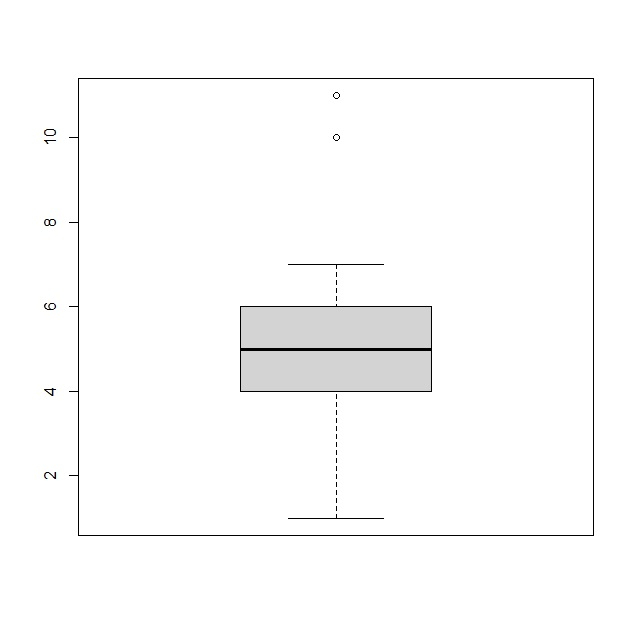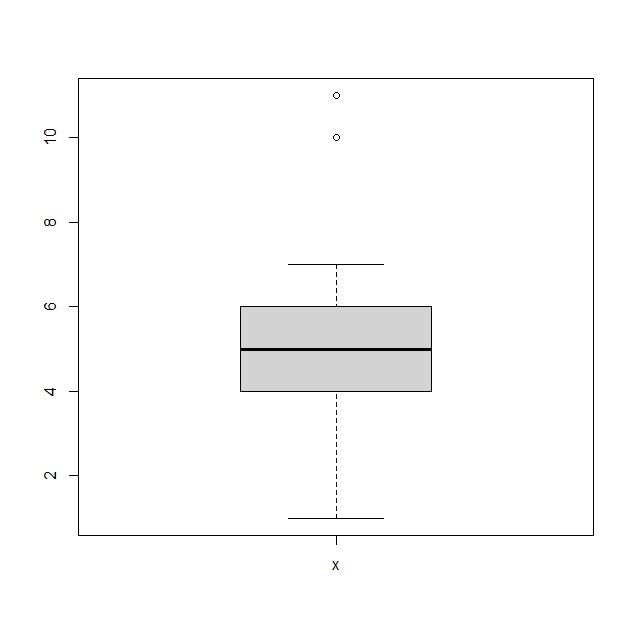# How to create a boxplot of single column in R data frame with column name?

To create a boxplot of single column in R data frame with column name, we can follow the below steps −

• First of all, create a data frame with single numerical column and create the boxplot for that column using boxplot function.

• Then, create the same boxplot with show.names argument set to TRUE.

## Example

#### Create the boxplot

Using boxplot function to create a boxplot for single column of a data frame as shown below −

x<-rpois(25,5)
df<-data.frame(x)
boxplot(df)

## OutputCreate the boxplot with column name

Create the boxplotsame as above with show.names argumentset to TRUE asshown below −

x<-rpois(25,5)
df<-data.frame(x)
boxplot(df,show.names=TRUE)

## Output